# Indirect Recursion

## Indirect Recursion in C Language with Example

In this article, I am going to discuss Indirect Recursion in C Language with Examples. Please read our previous article, where we discussed Tree Recursion with Examples.

##### Indirect Recursion in C

In Indirect recursion, there may be more than one function and they are calling one another in a circular fashion. For example, if the first function calls the second function, the second function calls the third function, and again the third function calls back the first function, then it becomes a cycle which is also a recursion i.e. Indirect Recursion.

For better understanding, please have a look at the below image. Here, we have a function called fun1, and if the condition inside the if block satisfies, then it calls another function called fun2. Similarly, inside function fun2, if the if condition satisfies then again it calls back to the function fun1. So, it becomes an Indirect Recursion. So, here, fun1 calls fun2 with the reduced value of an i.e. a-1, and fun2 calls fun1 with some reduced value of b i.e. b-3. So, they are calling each other and at some point, they may stop when the condition failed.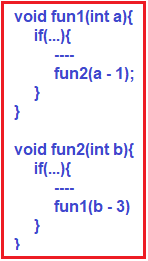So, in short, instead of function calling itself, it calls another function which again calls the first function. Let’s see a detailed explanation of Indirect Recursion with the tracing tree and understand this Indirect Recursion in a better way.

##### Example: Indirect Recursion in C Language:

In the below example, we have defined two functions fun1 and fun2. The fun1 function takes parameter a and checks if a is greater than 0, then it prints the value of a and then calls the function fun2 with the reduced value of “a” i.e. a – 1. Similarly, in function fun2, it is taking a parameter i.e. b. And it checks if b > 0, then it prints the value of b and then calls fun1 with the reduced value of b i.e. b-3. In this way both function calling each other until the condition fails.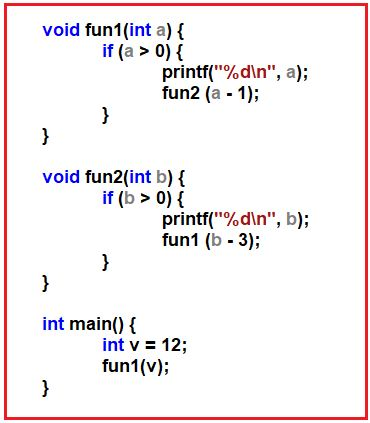#### Tracing Tree of Indirect Recursion:

Let us trace the above example step by step for a better understanding of how the Indirect Recursion works.

##### Step1: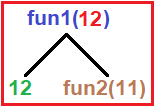Output: 12

Tracing Tree: In the above example, we call fun1 with a parameter of 12. First, the condition will check if 12 > 0? Yes, then it executes the next statement which is nothing but printing 12 on the screen. And the second statement is to call function fun2 with the parameter of 11 i.e. 12-1.

##### Step2: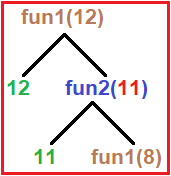Output: 12 11

Tracing Tree: Now the control goes to fun2 with a parameter of 11. First, it checks the condition if 11 > 0? Yes, then it executes the next statement which prints 11 on the screen. Then the second statement will execute which calls fun1 with a parameter of 8 i.e. 11-3.

##### Step3.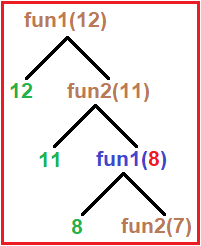Output: 12 11 8

Tracing Tree – In a circular manner, both the function calls each other until the condition fail. In this step, fun1 will receive the input parameter value as 8. Then it checks if 8 > 0? Yes, the condition satisfies. So, it executes the next statement which is nothing but printing 8 on the screen. And the second statement is to call function fun2 with the parameter of 7 i.e. 8-1.

##### Step4: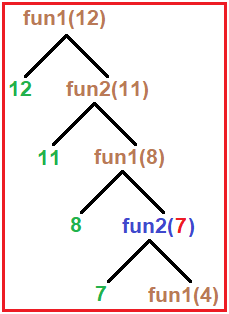Output: 12 11 8 7

Tracing Tree: Now the control goes to fun2 with a parameter of 7. First, it checks the condition if 7 > 0? Yes, the condition satisfies. So, it executes the next statement which prints 7 on the screen. Then the second statement will execute which calls function fun1 with a parameter of 4 i.e. 7-3.

##### Step5: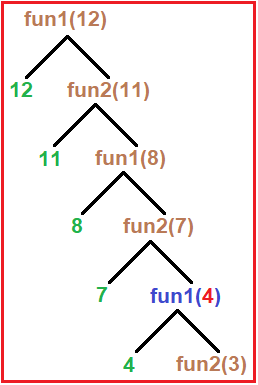Output: 12 11 8 7 4

Tracing Tree: Now, function fun1 with receive the input parameter value as 4. Then it checks if 4 > 0? Yes, the condition satisfies. So, it executes the next statement which is nothing but printing 4 on the screen. And the second statement is to call function fun2 with the parameter of 3 i.e. 4-1.

##### Step6: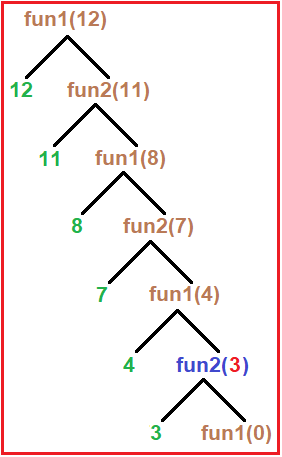Output: 12 11 8 7 4 3

Tracing Tree: Now the control goes to fun2 with a parameter of 3. First, it checks the condition if 3 > 0? Yes, the condition satisfies. So, it executes the next statement which prints 3 on the screen. Then the second statement will execute which calls function fun1 with a parameter of 0 i.e. 3-3.

##### Step7: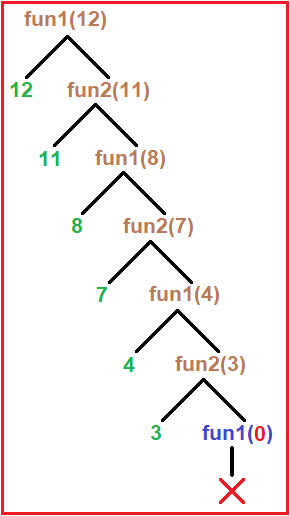Output: 12 11 8 7 4 3

Tracing Tree: Here fun1(0) will terminate, and no print and call statement will execute as the “if” condition fails here (0 > 0). From here control goes back to fun2(3) as it has also finished then control goes back from fun2(3) to fun1(4) and in the same reverse manner up to fun1(12) and you will get the output as 12 11 8 7 4 3. In this manner indirect recursion works.

##### Indirect Recursion Example in C Programming Language:
```#include <stdio.h>
void fun1(int a)
{
if (a > 0)
{
printf("%d\n", a);
fun2(a - 1);
}
}

void fun2(int b)
{
if(b > 0)
{
printf("%d\n", b);
fun1(b - 3);
}
}

int main ()
{
int v = 12;
fun1 (v);
}
```

Output: 12 11 8 7 4 3

Note: Let’s modify the above example and do a short analysis. In both the above function, they, first print the statement and then executes the call, but now we interchange these steps, which means first they execute the call and then print. Now, what should be the output? Now here printing will happen on the return time of functions. When the fun1(0) terminates then the printing starts as control goes back to the previous function as shown in the below image.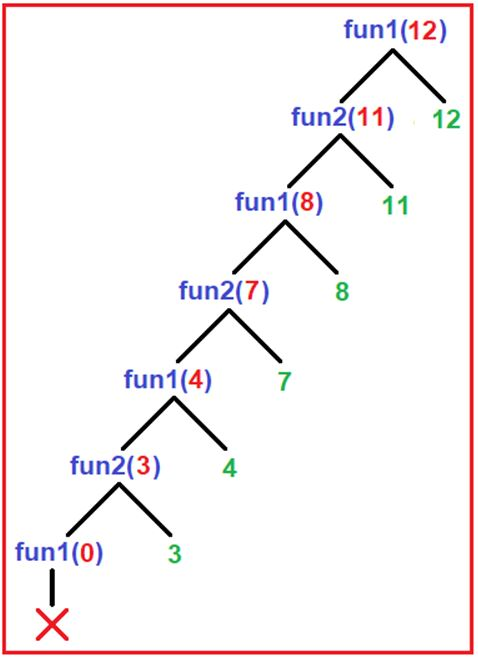##### Code:
```#include <stdio.h>
void fun1(int a)
{
if (a > 0)
{
fun2(a - 1);
printf("%d\n", a);
}
}

void fun2(int b)
{
if(b > 0)
{
fun1(b - 3);
printf("%d\n", b);
}
}

int main ()
{
int v = 12;
fun1 (v);
}
```

Output: 3 4 7 8 11 12

In the next article, I am going to discuss Nested Recursion in C Language with Examples. Here, in this article, I try to explain Indirect Recursion in C Language with Examples and I hope you enjoy this Indirect Recursion in C Language with Examples article.